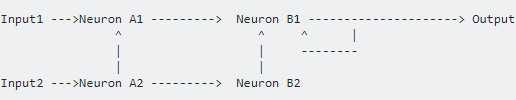2 views

I'm learning about artificial neural networks and have implemented a standard feed-forward net with a couple of hidden layers. Now, I'm trying to understand how a recurrent neural network(RNN) works in practice, and am having trouble with how activation/propagation flows through the network.

In my feed-forward, the activation is a simple layer by layer firing of the neurons. In a recurrent net, the neurons connect back to previous layers and sometimes themselves, so the way to propagate the network must be different. Trouble is, I can't seem to find an explanation of exactly how the propagation happens.

How might it occur say for a network like this:I imagined it would be a rolling activation with a gradual die down as the neuron's thresholds decrease the neuron firing to 0, much like in biology, but it appears there is a much more computationally efficient way to do this through derivatives?

by (108k points)

During forward propagation, a series of calculations are performed to generate a prediction and to calculate the cost. The cost is a function that we wish to minimize. Then, backpropagation calculates the gradient or the derivatives. This will be useful during the optimization phase because when the derivatives are close or equal to 0, it means that our parameters are optimized to minimize the cost function.

In a feed-forward, the propagation happens layer by layer, therefore Layer 1 neuron fire first, followed by Layers 2, 3, etc, so the propagation is one neuron activation stimulating activation in the neurons that take it as input.

Alternatively, we can think of propagation instead as the neurons whose inputs are active at any given point in time are the ones to fire. therefore if we have got a time t=0 were Layer 1 neurons are active, at the next time t=1 the next layer Layer 2 will activate since the neurons in Layer 2 take the neurons in Layer 1 as input.

If you want to know more about Neural Network visit this Neural Network Tutorial.